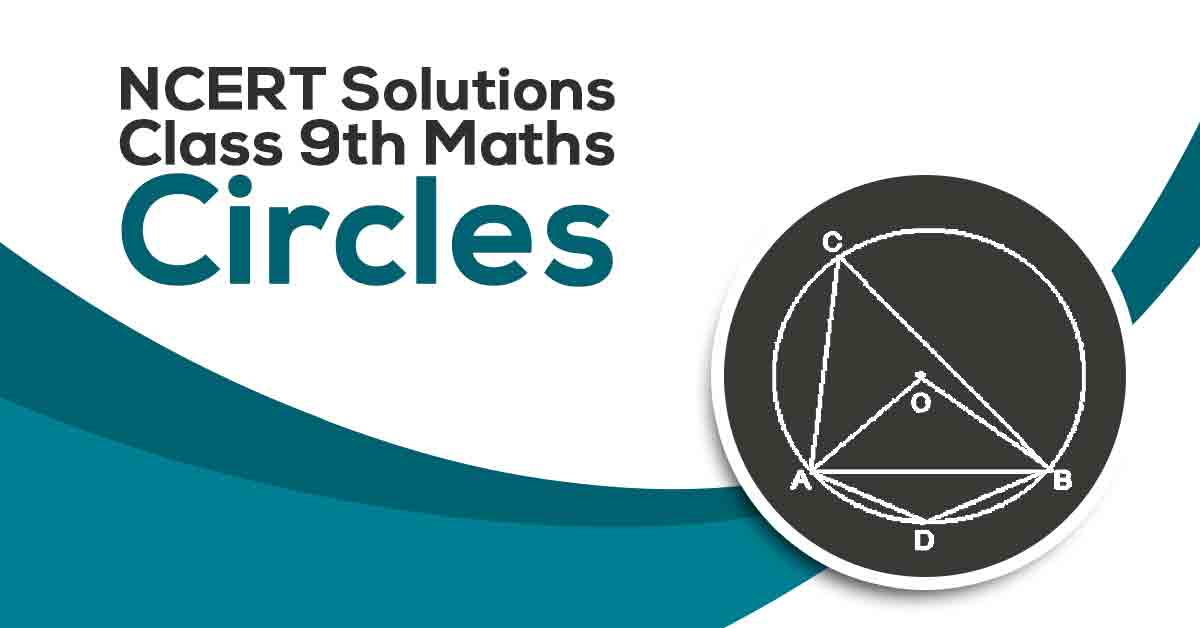NCERT Solutions for Class 9 Maths Chapter 10 | Vidyakul
×

# NCERT Solutions For Class 9 Maths Chapter 10

## NCERT Solutions For Class 9 Maths Chapter 10 Circles - PDF DownloadStudents require NCERT Solutions for Class 9 Maths Chapter 10 Circles for better exam preparation. In this chapter, we will learn that A circle is the collection of all points in a plane, which is equidistant from a fixed point in the plane. Equal chords of a circle (or of congruent circles) subtend equal angles at the center. If the angles subtended by two chords of a circle (or of congruent circles) at the center (corresponding centers) are equal, the chords are equal.

These CBSE NCERT Solutions for Class 9 Maths Chapter 10 Circles will help you to practice all the topics in the chapter with ease and score maximum marks in the examination.